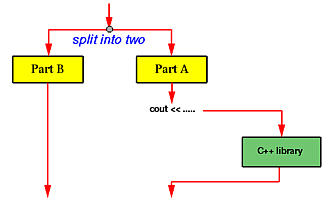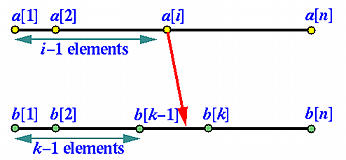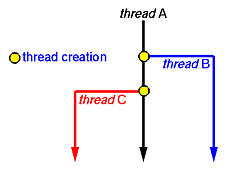### Archive

Archive for March, 2012

## Singleton Class

```using System;

public sealed class Singleton
{
private static volatile Singleton instance;
private static object syncRoot = new Object();

private Singleton() {}

public static Singleton Instance
{
get
{
if (instance == null)
{
lock (syncRoot)
{
if (instance == null)
instance = new Singleton();
}
}

return instance;
}
}
}```

This approach ensures that only one instance is created and only when the instance is needed. Also, the variable is declared to be volatile to ensure that assignment to the instance variable completes before the instance variable can be accessed. Lastly, this approach uses a syncRoot instance to lock on, rather than locking on the type itself, to avoid deadlocks.

This double-check locking approach solves the thread concurrency problems while avoiding an exclusive lock in every call to the Instance property method. It also allows you to delay instantiation until the object is first accessed.

Real World Example of a Singleton Class

Thread pools, SQL Connection Pools, Registry objects, Objects handling user preferences, Caches, Factory classes, Builder classes and Statistics utilities like a hit counter, log4net, when you call its logger, it uses a singleton class to return it.

Difference between Singleton and Static Class

Another question that usually comes up when it comes to using a Singleton is “Why not just use a static class?”. Static classes still have many uses and lots of times, people get confused and will use a Singleton as much as possible. One easy rule of thumb you can follow is if it doesn’t need to maintain state, you can use a Static class, otherwise you should use a Singleton.

So here is a quick list of uses for static classes:
Math.pow(double a, double b);
Interger.parseInt(String s);
Interger.toString(int i);

As you can see, the state of these methods don’t matter. You just want to use them to perform a simple task for you. But if you coding your application and you are using a central object where state does matter(such as the ModelLocator example), then its best to use a Singleton.

The next reason you may want to use a Singleton is if it is a particularly “heavy” object. If your object is large and takes up a reasonable amount of memory, you probably only one of those objects floating around. This is the case for things like a if you have a factory method that is particularly robust, you want to make sure that its not going to be instantiated multiple times. A Singleton class will help prevent such the case ever happening.

Categories: C# Tags:

Unfortunately, before you learn how to split your program into multiple execution threads, all programs you wrote contain a single execution thread. The following diagram shows an example. Suppose we have a program of two parts, Part A and Part B. After Part A finishes its computation, we use some cout statements to print out a large amount of output. As we all know, when a program prints, the control is transfered to a function in C++’s library and the execution of that program is essentially suspended, shown in dashed line in the diagram, until the printing completes. Once this (i.e., printing) is done, the execution of the program resumes and starts the computation of Part B. Is there anything wrong with this? No, we are used to it and we are trained to do programming in this way ever since CS101. However, is this way of programming good enough in terms of efficiency? It depends; but, in many situations, it is not good enough.If Part B must use some data generated by Part A, then Part B perhaps has to be executed after the output of cout completes. On the other hand, in many situations, Part A and Part B are independent of each other, or one may slightly rewrite both parts so that they do not depend on each other. In this case, Part B does not have to wait until cout completes. In fact, this is the key point! Therefore, before the execution of Part A, the program can be split into two execution threads, one for Part A and the other for Part B. See the diagram below. In this way, both execution threads share the CPU and all resources allocated to the program. Moreover, while Part A is performing the output which causes Part A to wait, Part B can take the CPU and executes. As a result, this version is more efficient than the previous one. Moreover, in a system with more than one CPUs, it is possible that the system will run both Part A and Part B at the same time, one on each CPU.In real programming practice, a program may use an execution thread for handling keyboard/mouse input, a second execution thread for handling screen updates, and a number of other threads for carrying out various computation tasks.

#### Example: Quicksort

The quicksort algorithm consists of two steps in each recursion. First, the partition step divides the input array segment into two segments such that all elements in the left segment is smaller or equal to all elements in the right segment. Second, the sorting step simply sorts the left segment and the right segment. After these two steps complete, the input segment is sorted. While it is not so obvious if the partition step can have multiple execution threads, one can split the execution of the sorting step into two, one for sorting the left segment while the other for sorting the right one. This is shown in the diagram below:#### Example: Merging

Consider another simple problem. Suppose we have two arrays a[ ] and b[ ] of n elements each. For simplicity, we assume that all of these 2n elements are different. Our job is to merge these two arrays into a sorted one. Everyone who took a data structures course knows how to do it; however, let us look at the same problem from a different angle.

Take an element from array a, say a[i]. We know that it is larger than i-1 elements of a. If we can figure out how many elements of b that are smaller than a[i], we will be able to know the exact location of a[i] in the sorted array. This is illustrated in the following diagram:With a slightly modified binary search, we can easily determine the location of a[i] in array b. There are only three possibilities:

1. a[i] is less than b: In this case, a[i] is larger than i-1 elements in a and smaller than all elements in b. Therefore, a[i] should be in position i of the sorted array.
2. a[i] is larger than b[n]: In this case, a[i] is larger than i-1 elements in a and n elements in b. Therefore, a[i] should be in position i+n of the sorted array.
3. a[i] is between b[k-1] and a[k]. In this case, a[i] is larger than i-1 elements in a and k-1 elements in b. Therefore, a[i] should be in position i+k-1 of the sorted array.

After the main program reads in both arrays, it can split itself into 2n execution threads, each of which handles an element in a or in b. Each of these execution threads determines its position in the merged array and writes the values into the corresponding location. After this, we will have a merged array! Thus, we use 2n threads, each of which takes O(log2(n)) comparisons to get the job done. In the conventional serial case, we use one execution thread which uses O(n) comparisons to merge the arrays.

#### Example: Matrix Multiplication

Another interesting application is the multiplication of two matrices. Suppose we have two matrices Am×k (m rows and k columns) and Bk×n (k rows and n columns) and want to compute the product of A and B into a matrix C of m rows and n columns. The entry of C on row i and column j is the sum of the products of the corresponding elements on row i of matrix A and column j of matrix B as shown below:How can we use multiple execution threads to solve this problem? We notice that the computation of Ci,j is independent of the computation of any other entries of matrix C. Because of this, after matrices A and B are read in, the main program can split m×n execution threads, one for each entry of matrix C. Each of these execution threads computes the products of the corresponding elements, sums them up, and stores the result into matrixC.

It requires k multiplications to compute a single entry of matrix C. Since there are m×n entries in C, the program with only one thread uses m×n×k multiplications. On the other hand, in the above scheme, each thread uses kmultiplications and there are m×n threads. If we have only one CPU, the multiple execution threads version may not be as efficient as the single execution thread one; however, if there are more than one CPUs, each of these CPUs may be assigned to a number of execution threads and the execution efficiency is higher. In the extreme case in which we have m×n CPUs to use, because all execution threads run at the same time, it only takes the time to compute one entry to complete the whole matrix multiplication. Thus, it is m×n times more efficient than the single execution thread version.The above examples may look a little unrealistic and their benefits seem only about program efficiency. There are other benefits of using multiple execution threads.In the matrix multiplication example, the main thread (i.e., the main program) must create a number of threads, one for each entry of the resulting matrix. A possibility is shown below. Two for statements are used to create m×n threads. We shall see more examples that dynamically create threads later.• When P executes a thread join in order to join with C, which is still running, P is suspended until C terminates. Once C terminates, P resumes.
• When P executes a thread join and C has already terminated, P continues as if no such thread join has ever executed (i.e., join has no effect).

A parent thread may join with many child threads created by the parent. Or, a parent only join with some of its child threads, and ignore other child threads. In this case, those child threads that are ignored by the parent will be terminated when the parent terminates.

Suppose you run a number of programs at the same time on a computer. It is possible that some CPU hogs keep eating up the CPU cycles so that other programs may hardly run. Well, this may be a problem of the scheduling policy of the operating system. However, when we write our programs with multiple threads, we have to make sure that some threads will not occupy the CPU forever, or for a very long time, without relinquishing it. Otherwise, we will end up in the situation where one or two threads keep running while the others simply wait there for their turns. That is, we run our threads in a very “polite” way that once a while a thread takes some rest so that the CPU can be used by other threads. This is achieved by thread yield.#### What is difference between Daemon and Non Daemon Thread

In java we have two type of Threads :

On the other hand we have Daemon threads. These threads are generally ‘Service provider’ threads. They should not be used to execute your program code but system code. These thread run in parallel to your code but JVM can kill them anytime. When JVM finds no user threads it stops and all daemon thread terminate instantly. Thus you should never depend on daemon thread to perform any program code.

Use events with thread; this is one of the techniques to synchronize one thread with other like `ManualResetEvent`

#### Difference between a computer process and thread

A single process can have multiple threads that share global data and address space with other threads running in the same process, and therefore can operate on the same data set easily. Processes do not share address space and a different mechanism must be used if they are to share data.

If we consider running a word processing program to be a process, then the auto-save and spell check features that occur in the background are different threads of that process which are all operating on the same data set (your document).

Process

In computing, a process is an instance of a computer program that is being sequentially executed by a computer system that has the ability to run several computer programs concurrently.

A single process may contain several executable programs (threads) that work together as a coherent whole. One thread might, for example, handle error signals, another might send a message about the error to the user, while a third thread is executing the actual task

#### Interlocked class

Provides atomic operations for variables that are shared by multiple threads. Interlocked class provides methods by which you can achieve following functionalities :-

1. increment Values.
2. Decrement values.
3.Exchange values between variables.